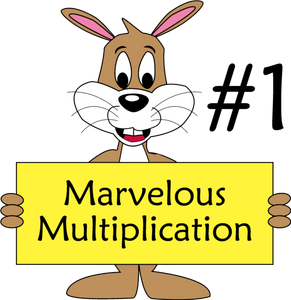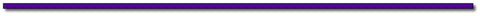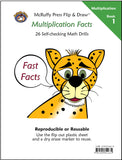# Marvelous Multiplication #1Marvelous Multiplication Trick #1: Using Math Squaring Tricks to Multiply Other Numbers.

If you haven’t already, check out our previous two blogs:

Quick Math: Find the Squares of Numbers That End With 5

Quick Math: Squaring Numbers 51 to 59

We can use these techniques to do some Quick Math multiplying two-digit numbers that aren't squares. The tricks in this post offer more of an entertainment value than everyday practical value. Helping children feel empowered to do math is a great thing. These tricks do that! Once after teaching these techniques to some children, they replied, “Wow! That’s what algebra does.”

Like the previous two tricks, the minimum a child has to understand is basic multiplication facts. The Why it Works section is great to help students who have some understanding of algebra see how math mysteries can be resolved.

The Trick:

We’ll start with the example of 64 x 66. After learning the trick you’ll immediately see that the product is 4224. First, let’s explore lists of problems we can use for the trick.

We can apply the trick to all these problems:

14 x 16, 24 x 26, 34 x 36, 44 x 46, 54 x 56, 64 x 66, 74 x 76, 84 x 86, 94 x 96

Do you see the pattern?

It also applies to these problems:

50 x 52, 51 x 53, 52 x 54, 53 x 55, 54 x 56, 55 x 57, 56 x 58, 57 x 59, 58 x 60, 59 x 61

And these problems:

19 x 21, 29 x 31, 39 x 41, 49 x 51, 59 x 61, 69 x 71, 79 x 81, 89 x 91

The lists of problems do not need to be memorized because there is a simple method to generate the problems:

Start with a number that the child has learned to easily square. We’ll call a number to be squared an Easy Square.

The problems were generated by adding and subtracting one from the numbers that were squared. For example, start with the Easy Square of 65.

Generate the problem by subtracting and adding one: (65 – 1)(65 + 1) = 64 x 66.

The solution is simply the product of the Easy Square minus 1. Applying the Quick Math trick from the first blog we can quickly find the solution. 4225 – 1 = 4224.

The middle row of problems was generated from the Easy Squares demonstrated in the second blog.

For example, using the trick we can quickly know that 532 = 2809. From that we generate the problem 52 x 54 = 2809 – 1 = 2808

We can call the squares of two-digit numbers ending with zero Easy Squares, too. That was how we generated the last row of problems with 9’s and 1’s in the units place.

For example: 802 = 6400, so 79 x 81 = 6400 – 1 = 6399.

Practice the trick, and we’ll explore how to greatly expand the list of problems that can be generated in our next blog.

The Teaching Sheet provides a form children can use to practice stepping through the process. Chose an easy square and write the digits in the boxes. Skip to the other side of the page and generate a -1, +1 problem. Use an easy square trick to find the square of the Easy Square. Write the answer in the Th, H, T, O boxes. Subtract 1 to find the product of the generated problem.

Why it Works:

Let’s build an algebra problem. We’ll represent the Easy Square with the letter a. We generated the multipliers by adding and subtracting one to an Easy Square.

(a – 1)(a + 1) = a2 + a – a – 12 =  a2 – 1

The algebra problem shows we can substitute any of our Easy Square numbers. Apply the easy square trick and subtract one.

The formula is not limited to two-digit numbers. For example:

2002 = 40,000    So 199 x 201 = 39,999

Nor is it limited to Easy Squares. It’s just more difficult to apply. For example generating a problem from 392:

38 x 40 = 392 -1  = 1521 – 1 = 1520. It’s actually easier to multiply 38 x 40 than to first square 39 and then subtract one, so the trick just isn’t helpful. Nevertheless, there is a trick involving this problem, but that’s for another blog.## Thanks for Visting Free Gift:

Offer Expires July 15, 2017Subscribe to this blog's RSS feed using http://feeds.feedburner.com/McruffyPress-McruffyMathBlog## McRuffy Press offers complete math curriculum by grade level, math activity books, manipulatives, games and more! Find more McRuffy Math products here.

Improve multiplication facts skills with our Multiplication Fast Fact books:Multiplication Facts (Book 1) Drills factors from 1 to 10 in our self-checking, non-consumable format that allows students to practice until they have mastered basic facts.Multiplication Practice (Book 2) Drills factors from 1 to 10 in our self-checking, non-consumable format that allows students to practice until they have mastered basic facts.